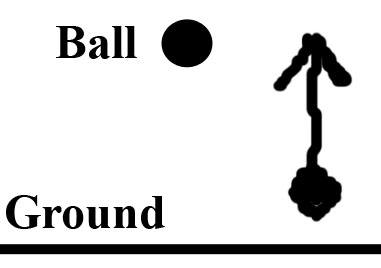# One-dimensional energy (potential/kinetic) problem

• Ascendant78

## Homework Statement

A 2kg ball is dropped from a height of 10m. Find its velocity when it reaches the ground. Define the positive direction on your motion diagram as going upwards (so as it head towards the ground, it is heading in the negative direction).

The problem itself was easy, but what our professor asked us that I am unsure about is where the negative sign is put into the equation to make the velocity a negative (based on the motion diagram).## Homework Equations

PEinitial = KEfinal

## The Attempt at a Solution

(2)(9.8)(10) = (1/2)(2)vfinal2
49 = vfinal2
v = 7m/s

Now, since the positive direction is supposed to be upwards, I am unsure as to what value should be made negative in the equation in order to make the 7m/s a negative value? Since g is causing a negative acceleration in regards to the motion diagram, I was tempted to make it a -9.8, but he told us last week that g is always positive regardless of direction.

I know in this problem it is easy to assess that v is negative based on the motion diagram, but he wanted us to think about this one because he said it will be important in more difficult problems later on.

## Homework Statement

A 2kg ball is dropped from a height of 10m. Find its velocity when it reaches the ground. Define the positive direction on your motion diagram as going upwards (so as it head towards the ground, it is heading in the negative direction).

The problem itself was easy, but what our professor asked us that I am unsure about is where the negative sign is put into the equation to make the velocity a negative (based on the motion diagram).## Homework Equations

PEinitial = KEfinal

## The Attempt at a Solution

(2)(9.8)(10) = (1/2)(2)vfinal2
49 = vfinal2
v = 7m/s

Now, since the positive direction is supposed to be upwards, I am unsure as to what value should be made negative in the equation in order to make the 7m/s a negative value? Since g is causing a negative acceleration in regards to the motion diagram, I was tempted to make it a -9.8, but he told us last week that g is always positive regardless of direction.

I know in this problem it is easy to assess that v is negative based on the motion diagram, but he wanted us to think about this one because he said it will be important in more difficult problems later on.

Velocity is a vector. Conservation of energy gave the square of the velocity, a positive quantity. The magnitude of the velocity is 7 m/s also positive. What is its the direction of the velocity, up or down? If "up" is positive, what should be the sign of the velocity?

If you use the kinematic equations to solve the problem, you will get the correct sign:

If y is the height above the ground, and the initial velocity is zero, y=10-gt2/2 and v=-gt.

ehild

Velocity is a vector. Conservation of energy gave the square of the velocity, a positive quantity. The magnitude of the velocity is 7 m/s also positive. What is its the direction of the velocity, up or down? If "up" is positive, what should be the sign of the velocity?

If you use the kinematic equations to solve the problem, you will get the correct sign:

If y is the height above the ground, and the initial velocity is zero, y=10-gt2/2 and v=-gt.

ehild

Thanks for the information. So what you are saying here is that it is fine to define g as -g if you know it is causing acceleration in the negative direction, correct?

So what you are saying here is that it is fine to define g as -g if you know it is causing acceleration in the negative direction, correct?
As your professor said, g is just a positive constant equal to 9.8 m/s^2. (It is the magnitude of the acceleration due to gravity.)

Depending upon your sign convention, the acceleration (which is downwards) can be +g or -g.

As your professor said, g is just a positive constant equal to 9.8 m/s^2. (It is the magnitude of the acceleration due to gravity.)

Depending upon your sign convention, the acceleration (which is downwards) can be +g or -g.

Ok, well that is easy enough. Thanks for the clarification.